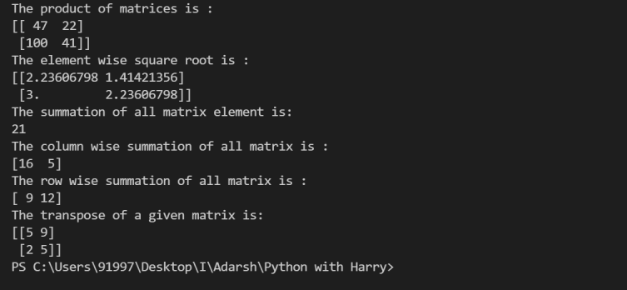### Python NumPy Matrix Manipulation Dot( ) and Transpose( )

What is the matrix?

A matrix is a two-dimensional data structure where numbers are arranged into rows and columns.

In python, the matrix can be used as a 2D List or 2D Array. Creating a matrix from the latter provides additional functionality for performing various tasks in the matrix. These functionality and configuration are defined in the "NumPy" module.

Now we will see various operations on Matrix in brief:

dot( ):- We use .dot( ) function to compute the matrix multiplication,rather than element wise multiplication.

sqrt( ) :- We use .sqrt( ) function to compute the square root of each element of matrix.

sum(x,axis) :- We use sum function to add all the elements in matrix.

{ 'axis' argument computes the column sum if axis is 0 and row sum if axis is 1}

T:- We use .T  argument  to transpose the given matrix.

Code:-

# Python code to demonstrate matrix operations
# dot(), sqrt(), sum() and "T"

# importing numpy for matrix operations
import numpy

# initializing matrices
x = numpy.array([[
5, 2], [9, 5]])
y = numpy.array([[
5, 4], [11, 1]])

# using dot() to multiply matrices
print ("The product of matrices is : ")
print (numpy.dot(x,y))

# using sqrt() to print the square root of matrix
print ("The element wise square root is : ")
print (numpy.sqrt(x))

# using sum() to print summation of all elements of matrix
print ("The summation of all matrix element is: ")
print (numpy.sum(y))

# using sum(axis=0) to print summation of all columns of matrix
print ("The column wise summation of all matrix is : ")
print (numpy.sum(y,axis=0))

# using sum(axis=1) to print summation of all columns of matrix
print ("The row wise summation of all matrix is : ")
print (numpy.sum(y,axis=1))

# using "T" to transpose the matrix
print ("The transpose of a given matrix is: ")
print (x.T)

Output:-